Get instant live expert help with Excel or Google Sheets“My Excelchat expert helped me in less than 20 minutes, saving me what would have been 5 hours of work!”

#### Post your problem and you’ll get expert help in seconds.

Your message must be at least 40 characters
Our professional experts are available now. Your privacy is guaranteed.

# Get fiscal year from date

A fiscal year is the period used for budget, accounting and financial reporting, and differs from a calendar year.  The fiscal year may vary among different countries and organizations. This step by step tutorial will assist all levels of Excel users in getting the fiscal year from a given date.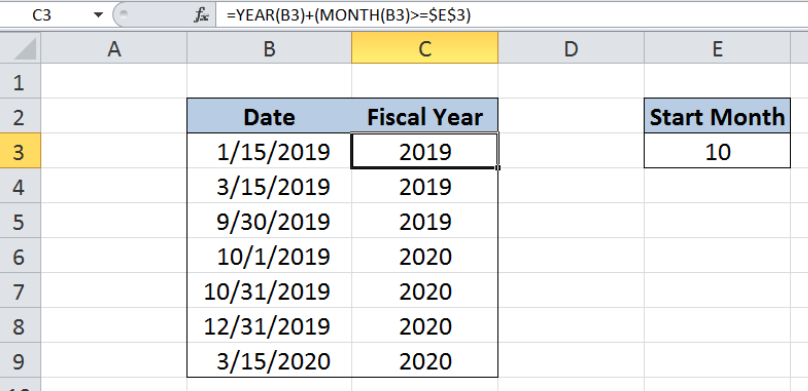Figure 1.  Final result: Get fiscal year from date

Final formula: `=YEAR(B6)+(MONTH(B6)>=\$E\$3)`

## Syntax of YEAR Function

YEAR function returns an integer corresponding to the year portion of a date; the year returned is between 1900 and 9999

`=YEAR(serial_number)`

• Serial_number – a date whose year we want to find; can be a date enclosed in quotation marks or a cell reference
• The date must be between January 1, 1900 and December 31, 9999

## Syntax of MONTH Function

MONTH function returns an integer corresponding to the month portion of a date; the value returned ranges from 1 (January) to 12 (December)

`=MONTH(serial_number)`

• Serial_number – a date whose month we want to find; can be a date enclosed in quotation marks or a cell reference
• The date must be between January 1, 1900 and December 31, 9999

## Setting up our Data

Our data consists of two columns: Date (column B) and Fiscal Year (column C).  In cell E3, we enter the value corresponding to the month that a fiscal year starts.  For this example, we enter the value 10, supposing that a fiscal year starts on October 1.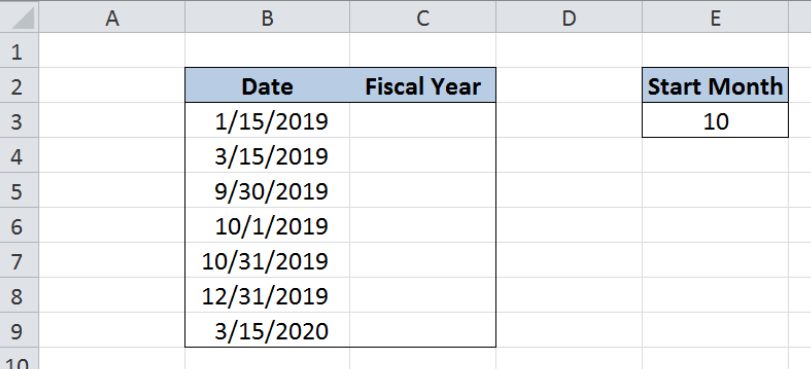Figure 2.  Sample data to get fiscal year from date

## Get fiscal year from date

Supposing that our fiscal year starts in October, then its period is from October 1 of the current year to September 30 of the succeeding year.

For every date entered in column B, we want to determine the fiscal year that it belongs to by using the YEAR and MONTH functions.  Let us follow these steps:

Step 1.  Select cell C3

Step 2.  Enter the formula: `=YEAR(B3)+(MONTH(B3)>=\$E\$3)`

Step 3.  Press ENTER

Step 4.  Copy the formula in C3 to cells C4:C9 by clicking the “+” icon at the bottom-right corner of cell C3 and dragging it down

The dollar symbol “\$” fixes cell E3 which serves as the reference for our formula to function properly across all cells in C3:C9.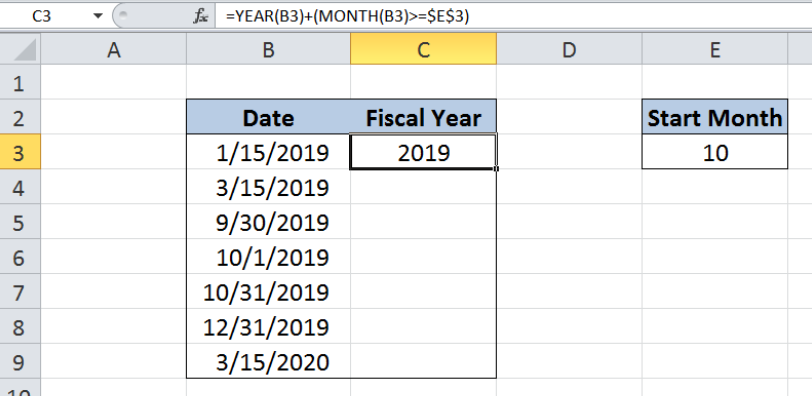Figure 3.  Entering the formula to get fiscal year from date

By standard, a fiscal year is represented by the year in which it ends.  Fiscal year 2019 will end on September 30, 2019, while the succeeding fiscal year 2020 will start on October 1, 2019.

YEAR function returns the year portion of the given date, while the MONTH function returns the numeric value for the month, where “1” refers to January and “12” refers to December.  This part of the formula (MONTH(B3)>=\$E\$3) returns the value “1” if TRUE, and “0” if FALSE.

Our formula extracts the year from the given date, then adds 1 if the date falls on or after the start month of 10.  Otherwise, it returns the same year as the given date.

Cells B3:B5 contain dates that fall before October 1, 2019.  As a result, the fiscal year returned is 2019.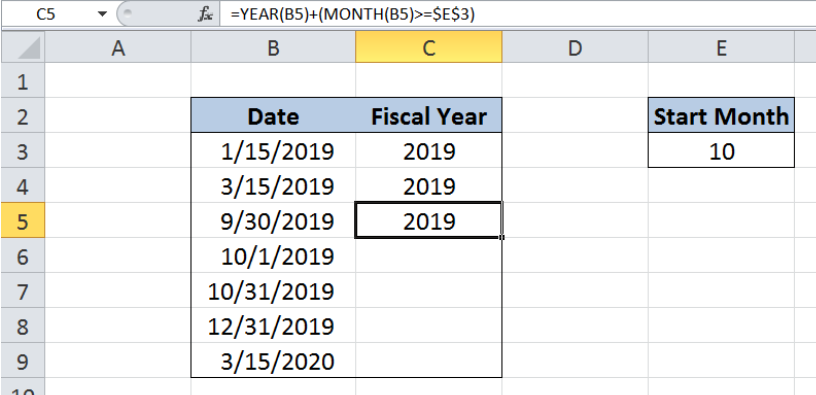Figure 4.  Dates before start month belonging to current fiscal year

For cells B6:B9, the dates fall on or after October 1, 2019.  Hence, these dates belong to the succeeding fiscal year, 2020.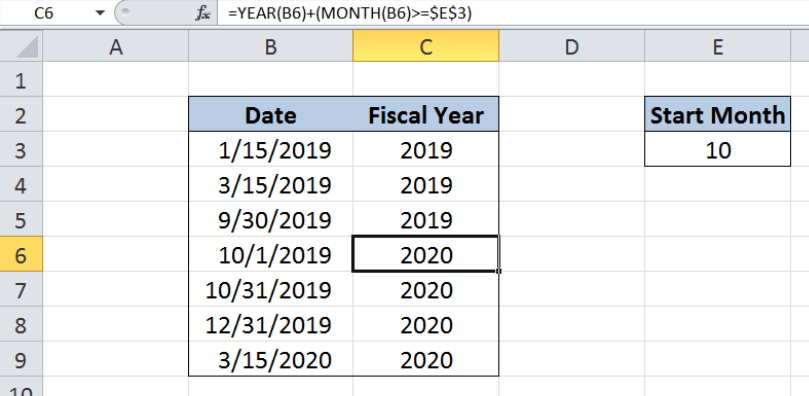Figure 5.  Output: Get fiscal year from date

Most of the time, the problem you will need to solve will be more complex than a simple application of a formula or function. If you want to save hours of research and frustration, try our live Excelchat service! Our Excel Experts are available 24/7 to answer any Excel question you may have. We guarantee a connection within 30 seconds and a customized solution within 20 minutes.

### Did this post not answer your question? Get a solution from connecting with the expert.Another blog reader asked this question today on Excelchat:
Solution examplesUse the Vlookup Function to complete the "employee" column of table 2. Use "job Id" from table 2 as your lookup_value(s) and table 1 as your reference.
Solved by C. H. in 16 minsIf a cell in another sheet is populated I need a vlookup done. If the cell is not populated I need the cell to return blank.
Solved by T. D. in 60 minsI am trying to make a chart that turns a week range red if nothing is entered in the range. If something is entered then I would like it to turn green. Please Help
Solved by E. U. in 43 minsI need a check box to show/hide an answer of an if function
Solved by Z. U. in 23 minsI need a formula to compare the data in two columns and then export the mismatched data in the 3rd column
Solved by S. Q. in 20 mins## Subscribe to Excelchat.coAnother blog reader asked this question today on Excelchat: